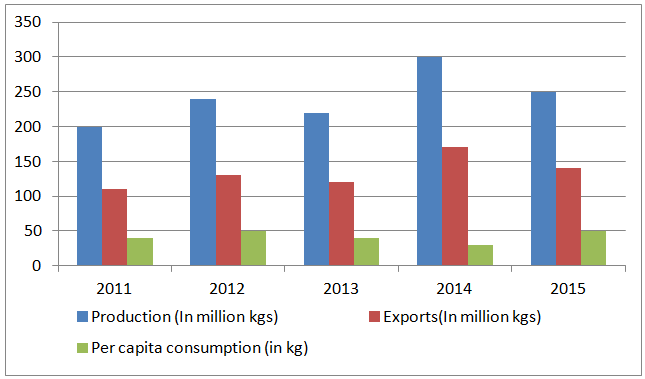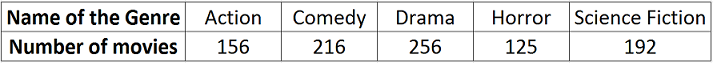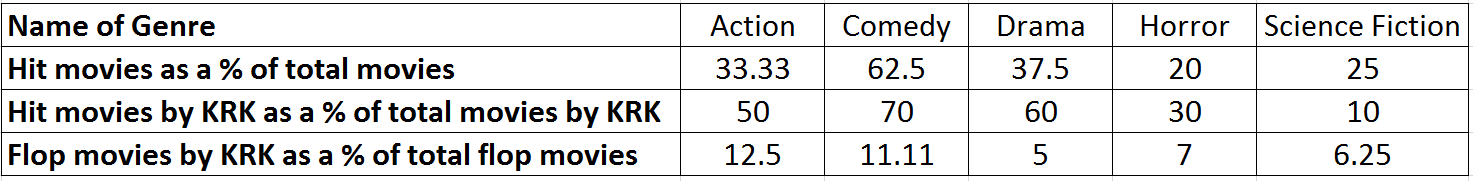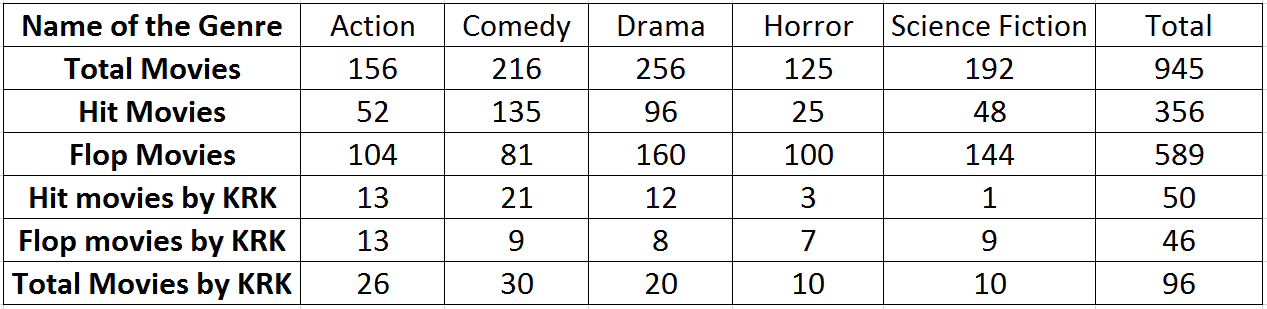# CAT Previous Year Data Interpretation Questions

1
7574

Data Interpretation (DI) is a crucial topic in the Data Interpretation and Logical Reasoning (LRDI) section of the CAT exam. To score well in this section, it is essential for CAT aspirants to be familiar with the types of DI questions that appear on the exam. One of the best ways to prepare for these questions is by reviewing previous years’ CAT DI questions. In this article, we will provide a comprehensive overview of CAT Previous Year Data Interpretation Questions, covering various question types and difficulty levels. By studying these questions, aspirants can get an idea of what to expect on the exam and develop strategies to solve them effectively.

CAT Data Interpretation Questions PDF

Instructions (1 – 5): Read the following information carefully and answer the questions which follow.

The following bar graph represents the production, exports and per capita consumption of wheat in Sri Lanka for five years 2011 to 2015.Consumption = Production – Exports
Per capita consumption = $$\frac{consumption}{population}$$

Question 1: The ratio of exports to consumption is highest for which year?

a) 2011
b) 2012
c) 2013
d) 2014

Question 2: Which year saw the highest percentage change in production?

a) 2012
b) 2013
c) 2014
d) 2015

Question 3: The population of Sri Lanka was highest in which year?

a) 2014
b) 2013
c) 2015
d) 2012

Question 4: What was the population of Sri Lanka in 2013

a) 2.5 million
b) 2.5 lacs
c) 3 million
d) 3 lacs

Question 5: The percentage change in consumption is highest for which year?

a) 2012
b) 2014
c) 2015
d) It was same for two years

Instructions (6 – 10): In a film industry every movie belongs to one of the five genres Action, Comedy, Drama, Horror and Science Fiction. Also, every movie falls in one category Hit or Flop.

Following table shows the number of movies from each genre released in this movie industry in the year 2015.Following table gives the hit and flop status of movies released in the year 2015 including those of a superstar called KRK.Question 6: What is the total number of hit movies of KRK across the genres Comedy, Drama and Horror in the year 2015?

a) 36
b) 39
c) 42
d) 46

Question 7: What percentage of the total movies that released in the year 2015 were flops?

a) 49.25%
b) 55.65%
c) 62.33%
d) 66.48%

Question 8: What percentage of the total movies that released in the year 2015 were flops?

a) 49.25%
b) 55.65%
c) 62.33%
d) 66.48%

Question 9: Across how many genres were the number of hits more than the number of flops for the actor KRK?

a) 1
b) 2
c) 3
d) 4

Question 10: How many movies of KRK were flops across the genres Action, Horror and Science fiction?
a) 16
b) 23
c) 29
d) None of these

Solutions for CAT Previous Year Data Interpretation Questions:

Solutions (1 – 5):

In 2011
Production = 200, Exports = 110 so consumption = 90
In 2012
Production = 240, Exports = 130 so consumption = 120
In 2013
Production = 220, Exports = 120 so consumption = 100
In 2014,
Production = 300, Exports = 170 so consumption = 130
In 2015
Production = 250, Exports = 140 so consumption = 110
Clearly the required ratio is highest for 2014.

In 2011
Production = 200
In 2012
Production = 240
In 2013
Production = 220
In 2014,
Production = 300
In 2015
Production = 250
So the percentage change in production is highest for the year 2014.

Population = Consumption/per capita consumption
In 2011
Consumption = 90, Per capita consumption = 40
In 2012
Consumption = 120, Per capita consumption = 50
In 2013
Consumption = 100, Per capita consumption = 40
In 2014,
Consumption = 130, Per capita consumption = 30
In 2015
Consumption = 110, Per capita consumption = 50
Clearly, this ratio is highest for the year 2014.

In 2013
Consumption = 100, Per capita consumption = 40
So required population = 100/40 = 2.5 million.

In 2011
Production = 200, Exports = 110 so consumption = 90
In 2012
Production = 240, Exports = 130 so consumption = 110
In 2013
Production = 220, Exports = 120 so consumption = 100
In 2014,
Production = 300, Exports = 170 so consumption = 130
In 2015
Production = 250, Exports = 140 so consumption = 110
So the percentage change in consumption is highest for the year 2014.

Solutions (6 – 10):

We fill the following table to answer this data interpretation set.
As we know the total number of movies in each category, we can find the hit movies in each category as the % given in the first row second table * number of movies.
Flop movies = Total Movies – Hit Movies
Flop movies by KRK = % given in 3rd row * Flop Movies
Total movies by KRK = Flop Movies / (1 – % given in second row)
Hit movies by KRK = Total movies by KRK – Flop movies by KRK